Duffing oscillator

This nonlinear oscillator is an example of a system which becomes chaotic when driven by a periodic force.

The oscillator itself is given by the equation:

x"(t)=-d*x(t)^3+e*x(t)-g*v(t)

The cubic term -d*x(t)^3 provides a nonlinear restoring force at large x, while the linear term pushes away from the origin. In addition, there is the usual velocity-proportional damping.

The potential for this oscillator has a double-well structure. At x =0 there is an unstable equilibrium point, and given some damping the particle has to fall into one side of the well or the other if it approaches the equilibrium point with just enough energy to move over it.

The homogeneous problem (non-driven oscillator) has no surprises in it. Given an initial condition there is a unique phase-space trajectory that leads to the particle winding up at the bottom of one of the two wells after the mechanical energy is converted to heat.

When the oscillator is driven by a periodic force the system can reach a limit cycle, where as much mechanical energy is lost per cycle as is dumped into the system by the crank. Chaos appears as a result of the two wells connected by the unstable equilibrium point.

> restart; unprotect(gamma):

> gamma:=1/10: eta:=1: delta:=1/4:

> plot(-1/2*eta*x^2+delta*x^4/4,x=-3..3,title="Potential Energy");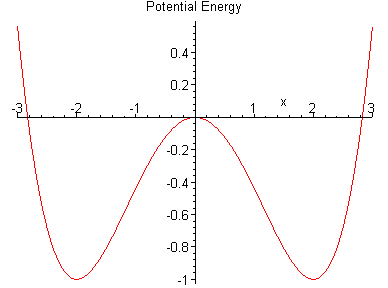> DE:=diff(x(t),t)=y(t),diff(y(t),t)=-gamma*y(t)+eta*x(t)-delta*x(t)^3;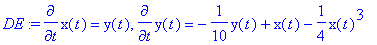We provide a solution example for the case where the oscillator initinally has sufficient energy to move across the unstable equilibrium point at the origin.

> IC:=x(0)=3,y(0)=10;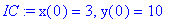> sol:=dsolve({DE,IC},{x(t),y(t)},numeric,output=listprocedure):

> X:=subs(sol,x(t)): Y:=subs(sol,y(t)):

> plot('X(t)',t=0..100,numpoints=500,title="Trajectory");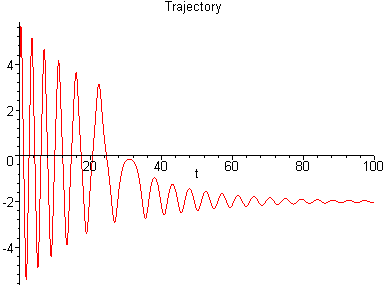The phase-space diagram illustrates how the loss of mechanical energy leads to the particle winding up in the x<0 well after five oscillations:

> plot(['X(t)','Y(t)',t=0..50],numpoints=500,title="Phase-space diagram");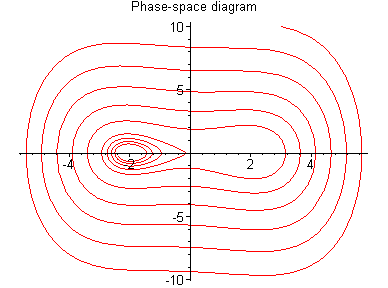Exercise 1:

Change the initial conditions and observe whether the particle gets trapped in the left-hand-side or right-hand-side well.

Driven case

Now we add a periodic driving force (amplitude 1 and circular frequency 1) and start the oscillator from the unstable equilibrium point:

> t:='t': nu:=1: DF:=sin(nu*t);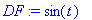> DE:=diff(x(t),t)=y(t),diff(y(t),t)=-gamma*y(t)+eta*x(t)-delta*x(t)^3+DF;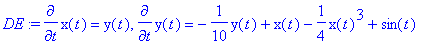> IC:=x(0)=0,y(0)=0; #start at the unstable equilibrium point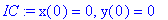> sol:=dsolve({DE,IC},{x(t),y(t)},numeric,output=listprocedure):

> X:=subs(sol,x(t)): Y:=subs(sol,y(t)):

> plot('X(t)',t=0..100,numpoints=500,title="Trajectory");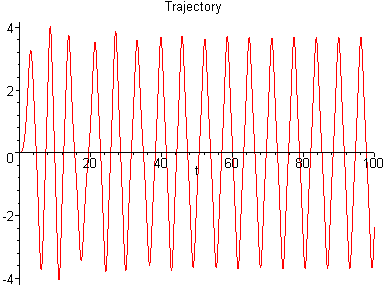> plot(['X(t)','Y(t)',t=0..50],numpoints=500,title="Phase-space Diagram");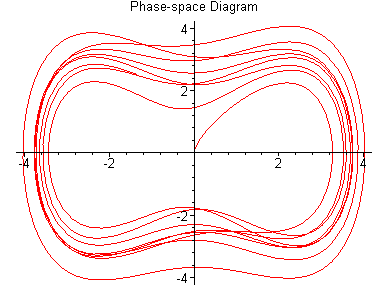Now we want the Poincare section: we accumulate a list of points by stroboscopically sampling with the driving force period:

> tau:=2*Pi/nu;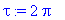> sol:=dsolve({DE,IC},{x(t),y(t)},numeric,method=lsode,output=listprocedure):

> X:=subs(sol,x(t)): Y:=subs(sol,y(t)):

> LL:=[[X(0),y(0)]]: for i from 1 to 1000 do: t_i:=i*tau; LL:=[op(LL),[X(t_i),Y(t_i)]]: od:

> plot(LL,style=point,symbol=box,symbolsize=4,axes=boxed,view=[-4..4,-4..4],title="Poincare Section");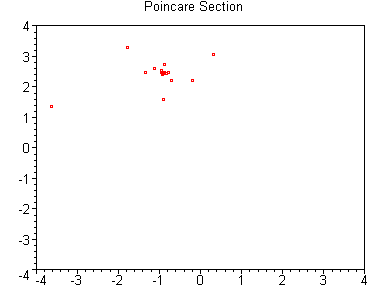What does this plot tell us?

For a simple limit cycle (asymptotically periodic motion with frequency of the driving force) we expect a single point: we catch the system at the same time during the cycle. Thus, we expect the Poincare points to cluster at a single point. In our case it happens to be somewhere inside the left-hand-side well with a positive velocity.

The few scattered point must have something to do with the transient behaviour before the limit cycle sets in.

Exercise 2:

Remove the first M points from the Poincare section, and confirm that the stroboscopic phase-space diagram with strobe frequency nu results in points which cluster around a single location in this case. Try for M =10,20,30,40 , and observe the Poincare section both on the large scale (-4 < x < 4, -4 < x' < 4), as well as on a fine scale (the vicinity of the accumulation point).

Now we double the frequency of the driving force. For the same amplitude as used before (1), we get a limit cycle inside one of the two wells. However, when we increase the amplitude rather interesting motion occurs:

> nu:=2: F0:=2.5: DF:=F0*sin(nu*t);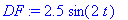> DE:=diff(x(t),t)=y(t),diff(y(t),t)=-gamma*y(t)+eta*x(t)-delta*x(t)^3+DF;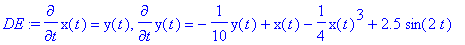> IC:=x(0)=0,y(0)=0; #start at the unstable equilibrium point> sol:=dsolve({DE,IC},{x(t),y(t)},numeric,output=listprocedure):

> X:=subs(sol,x(t)): Y:=subs(sol,y(t)):

> plot('X(t)',t=0..100,numpoints=2000,title="Trajectory");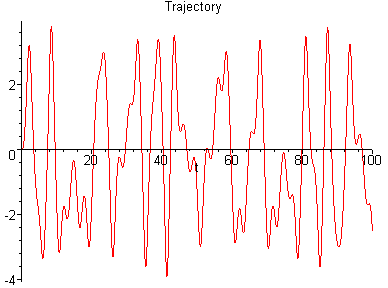> plot(['X(t)','Y(t)',t=0..50],numpoints=1000,title="Phase-space Diagram");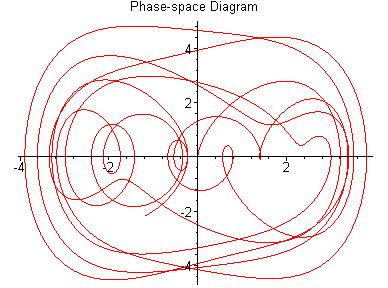The phase-space diagram is displayed with some small errors occasionally. Increasing the numpoints variable leads to more accuracy, but then the lines do not connect in same places, but are shown in dot form.

The Poincare section should be rather interesting now:

> tau:=2*Pi/nu;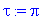> t:='t': sol:=dsolve({DE,IC},{x(t),y(t)},numeric,method=lsode,output=listprocedure):

> X:=subs(sol,x(t)): Y:=subs(sol,y(t)):

> LL:=[[X(0),Y(0)]]: for i from 1 to 1000 do: t_i:=i*tau; LL:=[op(LL),[X(t_i),Y(t_i)]]: od:

> plot(LL,style=point,symbol=box,symbolsize=4,axes=boxed,view=[-5..5,-8..4],title="Poincare Section");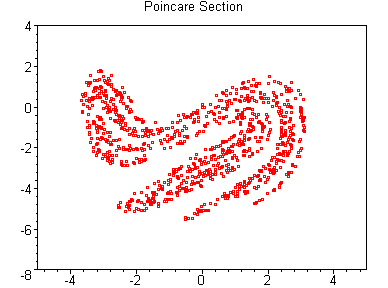The structure that appears in the Poincare section in this case can be proven to be a complicated curve, namely a fractal. This leads to the name 'strange attractor' for this oscillator, and is an indication that the system is chaotic (sensitive to intial conditions).

Exercise 3:

Change the parameters of the driving force (amplitude and frequency), and observe what happens to the Poincare section in these cases. Pick a frequency, and increase systematically the amplitude. Explore for which amplitude values F0 the Poincare diagram has a few discrete accumulation points, and for which values a multitude of points is observed (indicative of a strange attractor).

>